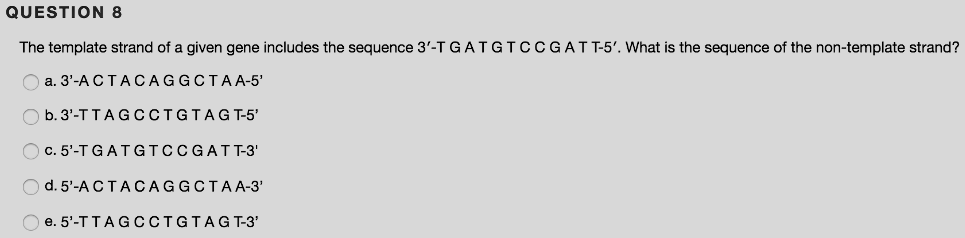# Problem: The template strand of a given gene includes the sequence 3' - T G A T G T C C G A T T - 5'. What is the sequence of the non-template strand?A. 3' - A C T A C A G G C T A A  - 5'B. 3' - T T A G C C T G T A G T - 5'C. 5' - T G A T G T C C G A T T - 3'D. 5' - A C T A C A G G C T A A - 3'E. 5' - T T A G C C T G T A G T - 3'

###### FREE Expert Solution

Nucleic Acids are polymers of nucleotides. These may either be single-stranded or double-stranded, due to the formation of H-bonds between the complementary bases of the two strands. In humans, DNA is in the double-stranded form.###### Problem Details

The template strand of a given gene includes the sequence 3' - T G A T G T C C G A T T - 5'. What is the sequence of the non-template strand?

A. 3' - A C T A C A G G C T A A  - 5'

B. 3' - T T A G C C T G T A G T - 5'

C. 5' - T G A T G T C C G A T T - 3'

D. 5' - A C T A C A G G C T A A - 3'

E. 5' - T T A G C C T G T A G T - 3'